JEE  >  JEE Main Physics Mock - 3

# JEE Main Physics Mock - 3

Test Description

## 25 Questions MCQ Test JEE Main & Advanced Mock Test Series | JEE Main Physics Mock - 3

JEE Main Physics Mock - 3 for JEE 2023 is part of JEE Main & Advanced Mock Test Series preparation. The JEE Main Physics Mock - 3 questions and answers have been prepared according to the JEE exam syllabus.The JEE Main Physics Mock - 3 MCQs are made for JEE 2023 Exam. Find important definitions, questions, notes, meanings, examples, exercises, MCQs and online tests for JEE Main Physics Mock - 3 below.
Solutions of JEE Main Physics Mock - 3 questions in English are available as part of our JEE Main & Advanced Mock Test Series for JEE & JEE Main Physics Mock - 3 solutions in Hindi for JEE Main & Advanced Mock Test Series course. Download more important topics, notes, lectures and mock test series for JEE Exam by signing up for free. Attempt JEE Main Physics Mock - 3 | 25 questions in 60 minutes | Mock test for JEE preparation | Free important questions MCQ to study JEE Main & Advanced Mock Test Series for JEE Exam | Download free PDF with solutions
 1 Crore+ students have signed up on EduRev. Have you?
JEE Main Physics Mock - 3 - Question 1

### The ratio of the energy of an X - ray photon of wavelength 1 Å to that of visible light of wave length 500 Å is

JEE Main Physics Mock - 3 - Question 2

### The de-Broglie wavelength associated with proton changes by 0.25% if its momentum is changed by P₀. The initial momentum was

Detailed Solution for JEE Main Physics Mock - 3 - Question 2 Use the formula lamba=h/p you will see that they are inversely related, so if you reduce wavelength by 0.25% then momentum reduces by 1 unit. So that implies Initial momentum is 401Po
JEE Main Physics Mock - 3 - Question 3

### A fixed volume of iron is drawn into a wire of length l. The extension x produced in this wire by a constant force F is proportional to

Detailed Solution for JEE Main Physics Mock - 3 - Question 3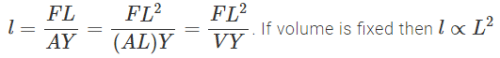JEE Main Physics Mock - 3 - Question 4

Condensers of capacities 2 μ F and 3 μ F are connected in series and a condenser of capacity 1 μ F is connected in parallel with them. The resultant capacity is

JEE Main Physics Mock - 3 - Question 5

Vapour is injected at a uniform rate in a closed vessel which was initially evacuated. The pressure in the vessel

Detailed Solution for JEE Main Physics Mock - 3 - Question 5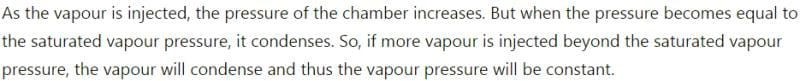JEE Main Physics Mock - 3 - Question 6

What is the coefficient of mutual inductance, when the magnetic flux changes by 2X10-2 Wb and change in current is 0.01 A ?

JEE Main Physics Mock - 3 - Question 7

The electric potential V is given as a function of distance x (metre) by V = (5x2 + 10x - 9) volt. Value of electric field at x = 1m is

Detailed Solution for JEE Main Physics Mock - 3 - Question 7

V = 5x2 + 10x - 9
E = - dv dx
= - (10x + 10)
= -(10 x 1 + 10)
= - 20 V/m

JEE Main Physics Mock - 3 - Question 8

If 3.8 x 10-6 is added to 4.2 x 10-5 giving due regard to significant figures, then the result will be

Detailed Solution for JEE Main Physics Mock - 3 - Question 8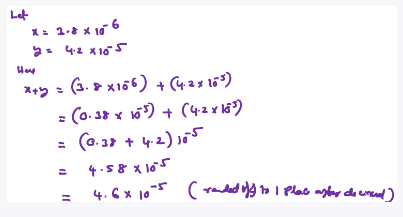JEE Main Physics Mock - 3 - Question 9

The mass and diameter of a planet have twice the value of the corresponding parameters of earth. Acceleration due to gravity on the surface of the planet is

JEE Main Physics Mock - 3 - Question 10

Referring to the above two questions, the acceleration due to gravity is given by

JEE Main Physics Mock - 3 - Question 11
Among electron, proton, neutron and $\alpha$ - particle the maximum penetration capacity is for
JEE Main Physics Mock - 3 - Question 12
In the following question, a Statement of Assertion (A) is given followed by a corresponding Reason (R) just below it. Read the Statements carefully and mark the correct answer-
Assertion(A): Pendulum of a clock is made of alloys and not of pure metals.
Reason(R): Use of alloys make the pendulum look good.
JEE Main Physics Mock - 3 - Question 13
In the following question, a Statement of Assertion (A) is given followed by a corresponding Reason (R) just below it. Read the Statements carefully and mark the correct answer-
Assertion(A): Lifting of aircraft is caused by pressure difference brought by varying speed of air molecules.
Reason(R): As the wings/aerofoils move against the wind, the streamlines crowd more above them than below, causing higher velocity above than below.
JEE Main Physics Mock - 3 - Question 14
The spectrum of light from an incandescent source is
Detailed Solution for JEE Main Physics Mock - 3 - Question 14 The spectrum of colors produced by incandescent lights is continuous, more similar to that which is produced by the sun, and incandescent lights transmit more red wavelengths than fluorescent light.
JEE Main Physics Mock - 3 - Question 15

The ratio of densities of nitrogen and oxygen is 14 : 16. The temperature at which the speed of sound in nitrogen will be same as that of oxygen at 55 oC is

Detailed Solution for JEE Main Physics Mock - 3 - Question 15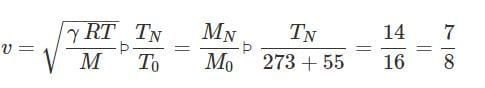p p TN=287K=14∘C
JEE Main Physics Mock - 3 - Question 16

The wavelength of ultrasonic waves in air is of the order of

JEE Main Physics Mock - 3 - Question 17
A bullet hits and gets embedded in a solid block resting on frictionless surface. In this process which one of the following is correct?
JEE Main Physics Mock - 3 - Question 18

Two rods of the same length and diameter having thermal conductivities K1 and K2 are joined in parallel. The equivalent thermal conductivity of the combination is

JEE Main Physics Mock - 3 - Question 19
A wave front travels
JEE Main Physics Mock - 3 - Question 20

The Figure shows a rectangular loop of resistance R, width l and length a being pulled at a constant speed vthrough a region of thickness d(>a)d>a in which a uniform magnetic field of induction BB acting into the plane of paper is set up. The position of the loop at instant t is indicated by x, the distance penetrated by the right edge of the loop into the field with x = 0 at t = 0. (see diagram). Ignore the fringe effects on the magnetic field on the loop.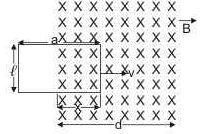Q. If a graph is plotted for the flux φB through the loop as a function of the coil position x, which of the following will show the correct graph?

*Answer can only contain numeric values
JEE Main Physics Mock - 3 - Question 21

If a radioactive material is decayed by 29.28% in 10 minute, then its half life will be:-

Detailed Solution for JEE Main Physics Mock - 3 - Question 21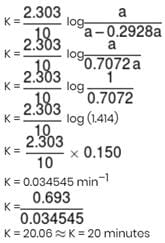*Answer can only contain numeric values
JEE Main Physics Mock - 3 - Question 22

The elevator shown in figure is descending with an acceleration of 2 ms–2. The mass of the block A = 0.5 kg. The force exerted by the block A on the block B is 2x (take g = 10 ms–2). find the value of x.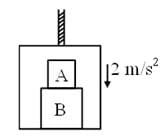Detailed Solution for JEE Main Physics Mock - 3 - Question 22

Let A applies force N1 on B
Then B also applies an opposite force N1 on A
As shown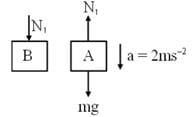For A    mg – N1 = ma
N1 = m(g – a) = 0.5 (10 – 2)
N1 = 4
N1 = 2x ⇒ x = 2

*Answer can only contain numeric values
JEE Main Physics Mock - 3 - Question 23

A Wheatstone's bridge is balanced with a resistance of 625 Ω in the third arm,  where P, Q and S are in the 1st, 2nd and 4th arm respectively. If P and Q are interchanged, the resistance in the third arm has to be increased by 51Ω to secure balance. The unknown resistance in the fourth arm is 6.5 × 10N Ω, then N will be :-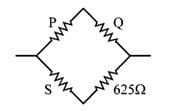Detailed Solution for JEE Main Physics Mock - 3 - Question 23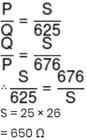*Answer can only contain numeric values
JEE Main Physics Mock - 3 - Question 24

If an objects is placed symmetrically between two plane mirrors, inclined at an angle of 72°, then the total number of images formed is:-

*Answer can only contain numeric values
JEE Main Physics Mock - 3 - Question 25

Two tuning forks A and B vibrating simultaneously produce 5 beats. Frequency of B is 512. It is seen that if one arm of A is filed, then the number of beats increases. Frequency of A will be:-

Detailed Solution for JEE Main Physics Mock - 3 - Question 25

After filling frequency increases, so nincreases(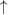­). Also it is given that beat frequency increases (i.e., x­)
Hence nA­– nB = x­   ...(i)               → Correct
nB – nA­= x­...(ii)             → Wrong
⇒ nA = nB + x = 512 + 5 = 517 Hz.

## JEE Main & Advanced Mock Test Series

2 videos|324 docs|165 tests
 Use Code STAYHOME200 and get INR 200 additional OFF Use Coupon Code
Information about JEE Main Physics Mock - 3 Page
In this test you can find the Exam questions for JEE Main Physics Mock - 3 solved & explained in the simplest way possible. Besides giving Questions and answers for JEE Main Physics Mock - 3, EduRev gives you an ample number of Online tests for practice

## JEE Main & Advanced Mock Test Series

2 videos|324 docs|165 tests